Using Spreadsheets to Simulate Process Dynamics -
A Short Tutorial

I. Euler Method for Solving Initial Value Problems

Given: a differential equation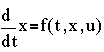the function u(t), and initial condition x(0)

Required: a series of number-pairs,

(t0,x0) , (t1,x1) , (t2,x2) ,  , (tN,xN)

such that for 0 £ k £ N
xk = x ( t = tk )

approximate the solution of the given differential equation. Method:
1. Let t0, t1, , tN be evenly spaced, i.e. tk=k Dt.

2.
3. If Dt is sufficiently small, the derivative dx/dt could be approximated by the finite difference

4.

dx/dt »Dx/Dt

where Dx = xk+1 - xk

5. Now substitute these approximations to the original differential equation to obtain

6.

dx/dt »Dx/Dt = f(tk,xk,uk)

xk+1-xk=Dt f(tk,xk,uk)

xk+1=xk+Dt f(tk,xk,uk)

where we have set the evaluation of the function f at t=tk, and so uk is just u(t=tk).

The last equation above is known as the recursion equation, also known as the finite difference approximation. All terms on the left-hand side of the recursion equation are known (current values used). Evaluating the left-hand side yields (predicts) the next value of x in the series.

7. Start the simulation by using the initial conditions, i.e. k=0,

8. x1 = x0 + Dt f(0,x0,u0)

9. Continue until we get xN, i.e.
x2 = x1 + Dt f( Dt, x1, u1 )

x3 = x2 + Dt f( 2Dt, x2, u2 )

xN = xN-1 + Dt f( (N-1)Dt, xN-1, uN-1)

( you can then plot xk vs. tk )

For discussion purposes, suppose we have the process model for liquid level change in a cylindrical tank.
dh/dt = (Fin-Fout)/A
where Fin is the volumetric flow rate into the tank, Fout=kv h1/2 is the volumetric flow rate out of the tank (behaving according to Torricelli's law) with kv as valve coefficient, and A is the cross-sectional area.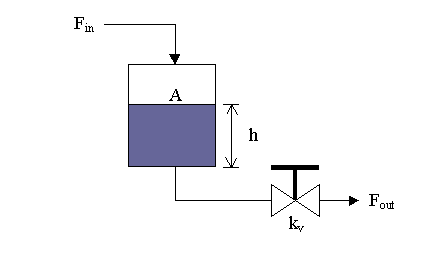The main purpose of a simulation is to observe changes in the behavior when certain parameters of the system are tweaked.

For our scenario, let us choose the following settings:

Fin=1 ft3/sec

kv=1.25 ft2.5

A=0.75 ft2

and we will treat kv and A as parameters. For our initial condition, let h(0)=1 ft.

To compare our case with the discussion of Eulers method above, we have x=h , u=Fin , and

f( t, x, u )=f( t, h, Fin )=( Fin - kvh1/2 ) /A

so the recursion equation is given by

hk+1 = hk + Dt ( Fin,k - kvhk1/2 )/A

Now, let us implement this in a spreadsheet (figures below were generated using Microsoft Excel):

1. First, lay out the constants ( say Dt=0.1) and parameters, and fill-in the time column. Also, you can fill-in Fin values and the h0 value.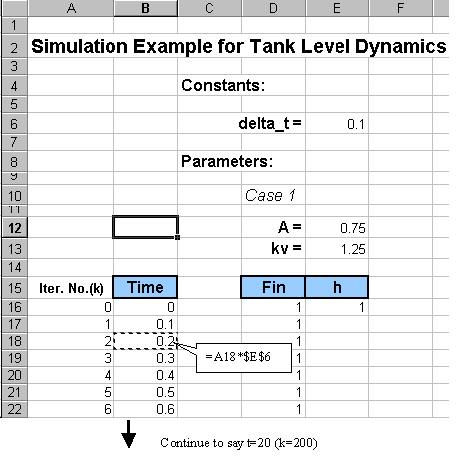2. Plug in the recursion formula for h1 and copy the formula to the cells below.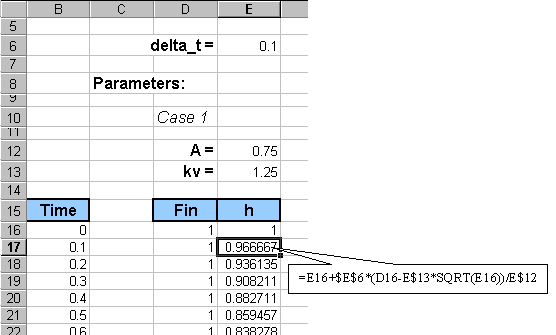Take note of the absolute and relative cell addressing. We chose to use the address E\$12 for A and address E\$13 for kv because we are planning to copy the block from cell D10 to E216 to a location to the left, to begin another case study.

3. Plot h vs time.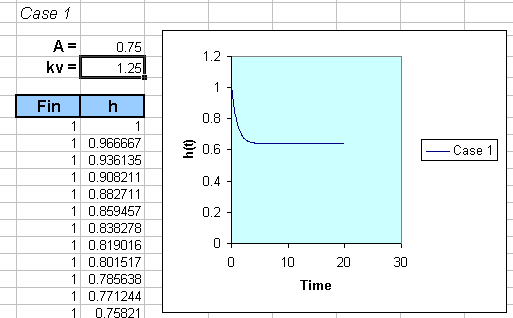You could actually change the value of A in cell E12 and then observe how the response will change accordingly.

4. As mentioned earlier, to investigate how the response will behave to a set of parameter changes, we can collect several cases by copying the block from cell D10 to E216 to another location and then change one of the parameters, say A below: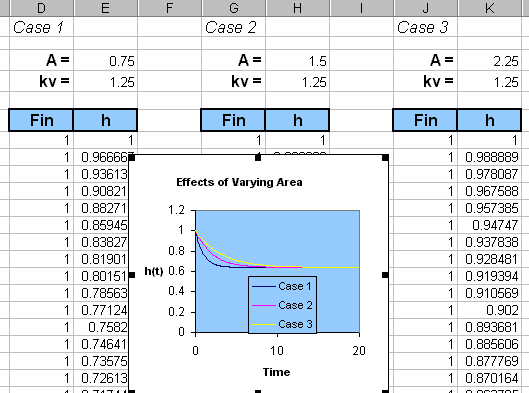5. As another example, suppose instead of Fin(t)=1.0, we decide to investigate a case where
Fin= 1 when t<10, and Fin=2 when t ³ 10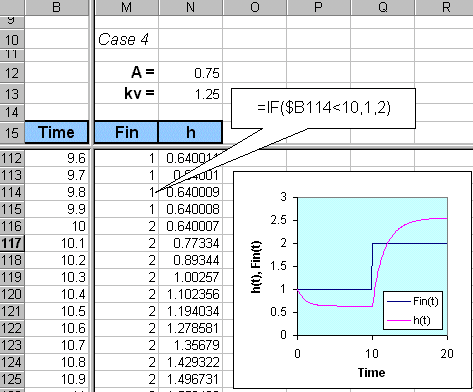III. Higher Order Differential Equations
We will limit the discussion to second order, but the pattern should hold for orders greater than 2.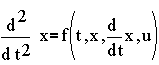We first need to introduce new variables to denote the derivatives. Let v = dx/dt, then we can reduce the original second order equation to a set of 2 first order equations given by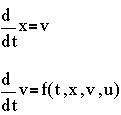Following the same approach as before of approximating derivatives by finite differences, we get two recursion equations

xk+1 = xk + Dt vk

vk+1 = vk + Dt f(tk,xk,vk,uk)

The simulation will be initialized by conditions x0=x(0) and v0= dx/dt(0).

This page is maintained by Tomas B. Co (tbco@mtu.edu). Last revised 12/2/98.

Tomas B. Co
Associate Professor
Department of Chemical Engineering
Michigan Technological University
1400 Townsend Avenue
Houghton, MI 49931-1295